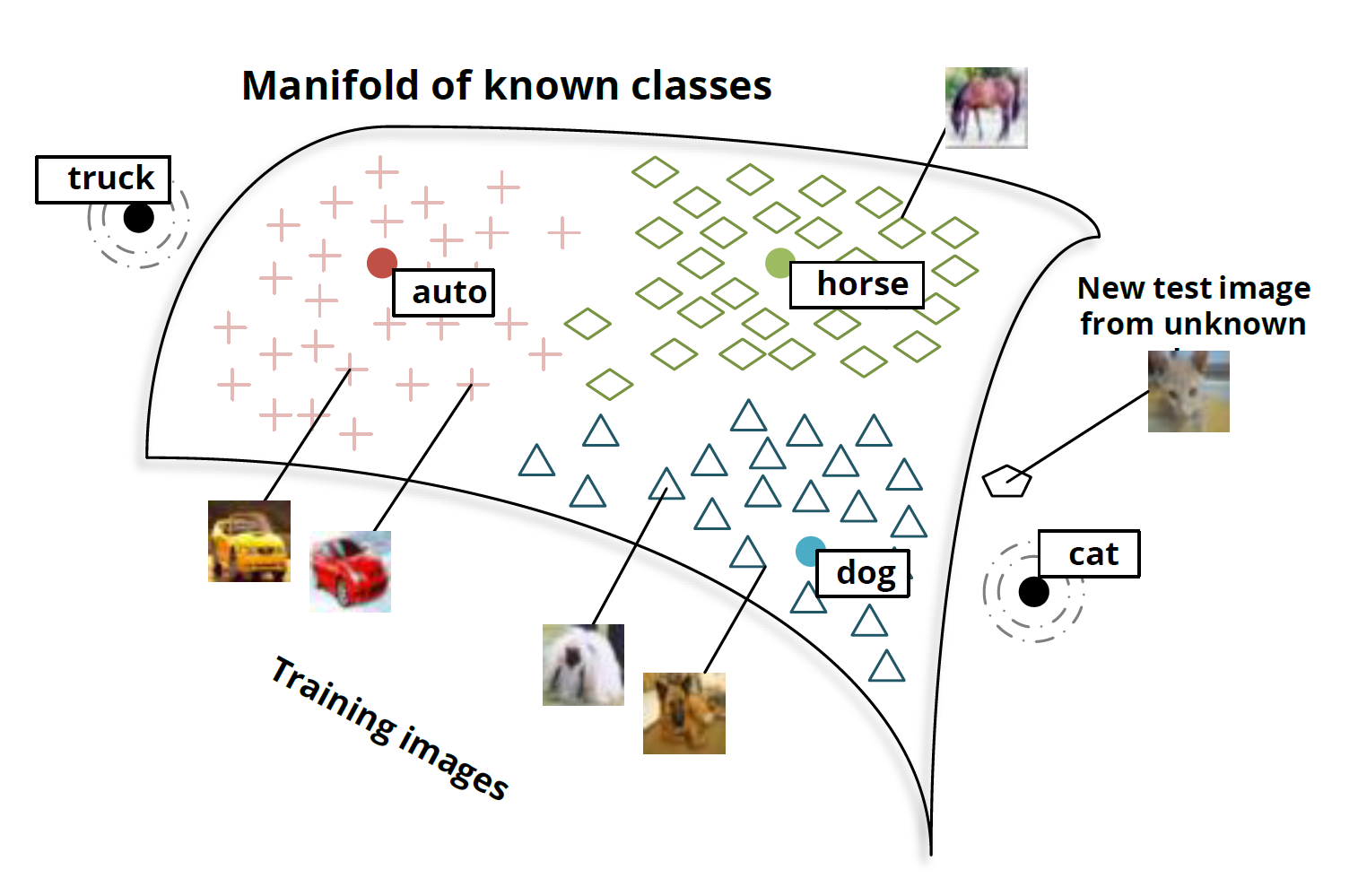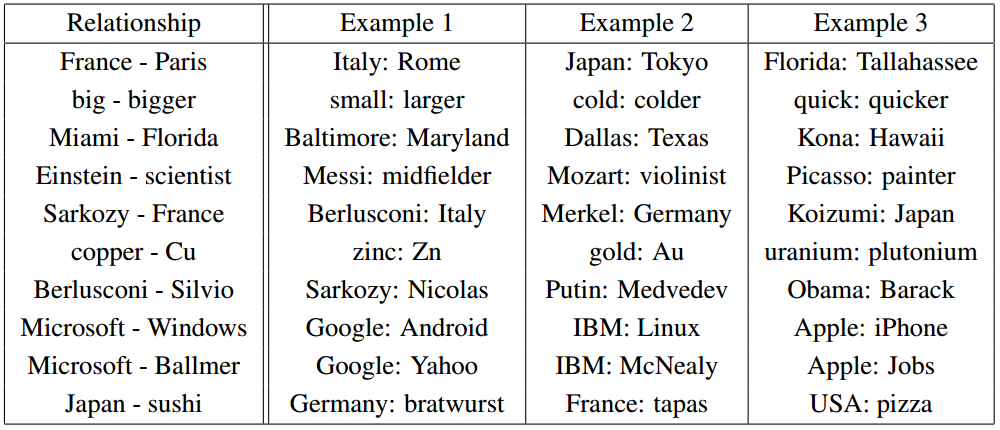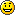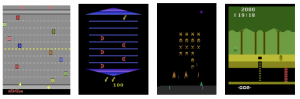# Neural Nets

You are currently browsing the archive for the Neural Nets category.

## The Best Deep Learning Blog Post Ever (Christopher Olah)Christopher Olah wrote an incredibly insightful post on Deep Neural Nets (DNNs) titled “Deep Learning, NLP, and Representations“.  In his post, Chris looks at Deep Learning from a Natural Language Processing (NLP) point of view.  He discusses how many different deep neural nets designed for different NLP tasks learn the same things.   According to Chris and the many papers he cites, these DNNs will automatically learn to intelligently embed words into a vector space.  Words with related meanings will often be clustered together.  More surprisingly, analogies such as “France is to Paris as Italy is to Rome” or “Einstein is to scientist as Picasso is to Painter” are also learned by many DNNs when applied to NLP tasks.  Chris reproduced the chart of analogies below from “Efficient Estimation of Word Representations in Vector Space” by Mikolov, Chen, Corrado, and Dean (2013).Relationship pairs in a word embedding. From Mikolov et al. (2013).

Additionally, the post details the implementation of recurrent deep neural nets for NLP.  Numerous papers are cited, but the writing is non-technical enough that anyone can gain insights into how DNNs work by reading Chris’s post.

So why don’t you just read it like NOW  — CLICK HERE.## Linear-Nonlinear-Poisson Neurons

The linear-nonlinear-Poisson (LNP) cascade model is a standard model of neuron responses.  Louis Shao has recently shown that an artificial neural net consisting of LNP neurons can simulate any Boltzmann machine and perform “a semi-stochastic Bayesian inference algorithm lying between Gibbs sampling and variational inference.”  In his paper he notes that the “properties of visual area V2 are found to be comparable to those on the sparse autoencoder networks ; the sparse coding learning algorithm  is originated directly from neuroscience observations; also psychological phenomenon such as end-stopping is observed in sparse coding experiments .”

## “A Neuro-evolution Approach to General Atari Game Playing”In “A Neuro-evolution Approach to General Atari Game Playing“, Hausknecht, Miikkulainen, and Stone (2013) describe and test four general game learning AIs based on evolving neural nets. They apply the AIs to sixty-one Atari 2600 games exceeding the best known human performance in three of the sixty-one games (Bowling, Kung Fu Master, and Video Pinball).  This work improves their previous Atari gaming AI described in “HyperNEAT-GGP: A HyperNEAT-based Atari General Game Player” (2012) with P. Khandelwal.

The Atari 2600 presents a uniform interface for all its games:  a 2D screen, a joystick, and one button.  The Atari 2600 games are simulated in the Arcade Learning Environment which has allowed several researchers to develop AIs for the Atari.

The four algorithms tested are:

1. Fixed topology neural nets that adapt by changing weights between neurons
2. Neural nets that evolve both the weights and the topology of the network (NEAT created by Stanley and Miikkulainen (2002))
3. “Indirect encoding of the network weights”  (HyperNEAT created by Gauci and Stanley (2008))
4. “A hybrid algorithm combining elements of both indirect encodings and individual weight evolution” (HybrID by Clune, Stanley, Pennock, and Ofria (2011))

All of the algorithms evolved a population of 100 individual neural nets over 150 generations mostly using the topology shown below.##### For MOVIES of the AIs in action click below

http://www.cs.utexas.edu/~mhauskn/projects/atari/movies.html

## “Resurgence in Neural Networks”

T Jake Luciani wrote a nice, easy to read blog post on the recent developments in neural networks.

## “Autoencoders, MDL, and Helmholtz Free Energy”

In “Autoencoders, MDL, and Helmholtz Free Energy“, Hinton and Zemel (2001) use Minimum Description Length as an objective function for formulating generative and recognition weights for an autoencoding neural net.  They develop a stochastic Vector Quantization method very similar to mixture of Gaussians where each input vector is encoded with

$$E_i = – \log \pi_i – k \log t + {k\over2} \log 2 \pi \sigma^2 + {{d^2} \over{2\sigma^2}}$$

nats (1 nat = 1/log(2) bits = 1.44 bits) where $t$ is the quantization width, $d$ is the Mahalanobis distance to the mean of the Gaussian, $k$ is the dimension of the input space, $\pi_i$ is the weight of the $i$th Gaussian.  They call this the “energy” of the code.  Encoding only using this scheme wastes bits because, for example, there may be vectors that are equally distant from two Gaussian. The amount wasted is

$$H = -\sum p_i \log p_i$$

where $p_i$ the probability that the code will be assigned to the $i$th Gaussian. So the “true” expected description length is

$$F = \sum_i p_i E_i – H$$

which “has exactly the form of the Helmholtz free energy.”  This free energy is minimized by setting

$$p_i = {{e^{-E_i}}\over{\sum_j e^{-E_j}}}.$$

In order to make computation practical, they recommend using a suboptimal distributions “as a Lyapunov function for learning” (see Neal and Hinton 1993). They apply their method to learn factorial codes.

## “Quantum information and the Brain”

Scott Aaronson gave a wonderful talk at NIPS 2012 called “Quantum information and the Brain“.  I really enjoyed his very concise intuitive description of quantum mechanics and his sceptical yet fair attitude towards the ideas expressed by Penrose in “The Emperor’s New Mind”.

## Bengio LeCun Deep Learning Video

Bengio and Lecun created this wonderful video on Deep Neural Networks.  Any logical function can be represented by a neural net with 3 layers (one hidden, see e.g. CNF), however simple 4 level logical functions with a small number of nodes may require a large number of nodes in a 3 layer representation.  They point to theorems that show that the number of nodes required to represent a k level logical function can require an exponential number of nodes in a k-1 level network. They go on to explain denoising auto encoders for the training of deep neural nets.

## Dropout – What happens when you randomly drop half the features?

In “Improving neural networks by preventing co-adaptation of feature detectors“, Hinton, Srivastava, Krizhevsky, Sutskever, and Salakhutdinov answer the question:  What happens if “On each presentation of each training case, each hidden unit is randomly omitted from the network with a probability of 0.5, so a hidden unit cannot rely on other hidden units being present.”  This mimics the standard technique of training several neural nets and averaging them, but it is faster.  When they applied the “dropout” technique to a deep Boltzmann neural net on the MNIST hand written digit data set and the TIMIT speech data set, they got robust learning without overfitting.  This was one of the main techniques used by the winners of the Merck Molecular Activity Challenge.

Hinton talks about the dropout technique in his video Brains, Sex, and Machine Learning.

## Kurweil’s book on Creating a Mind

Check out the Bookworm9765’s review of Kurzweil‘s book “How to Create a mind” on Amazon.com.  Here is a snippet:

The core of Kurzweil’s theory is that the brain is made up of pattern processing units comprised of around 100 neurons, and he suggests that the brain can be understood and simulated primarily by looking at how these lego-like building blocks are interconnected.

## Book “How to Build a Brain”

It looks like Canadian Professor and Director, Centre for Theoretical Neuroscience Chris Eliasmith is having some success constructing “the world’s largest simulation of a functioning brain.”  His book titled “How to Build a Brain” expected in February.